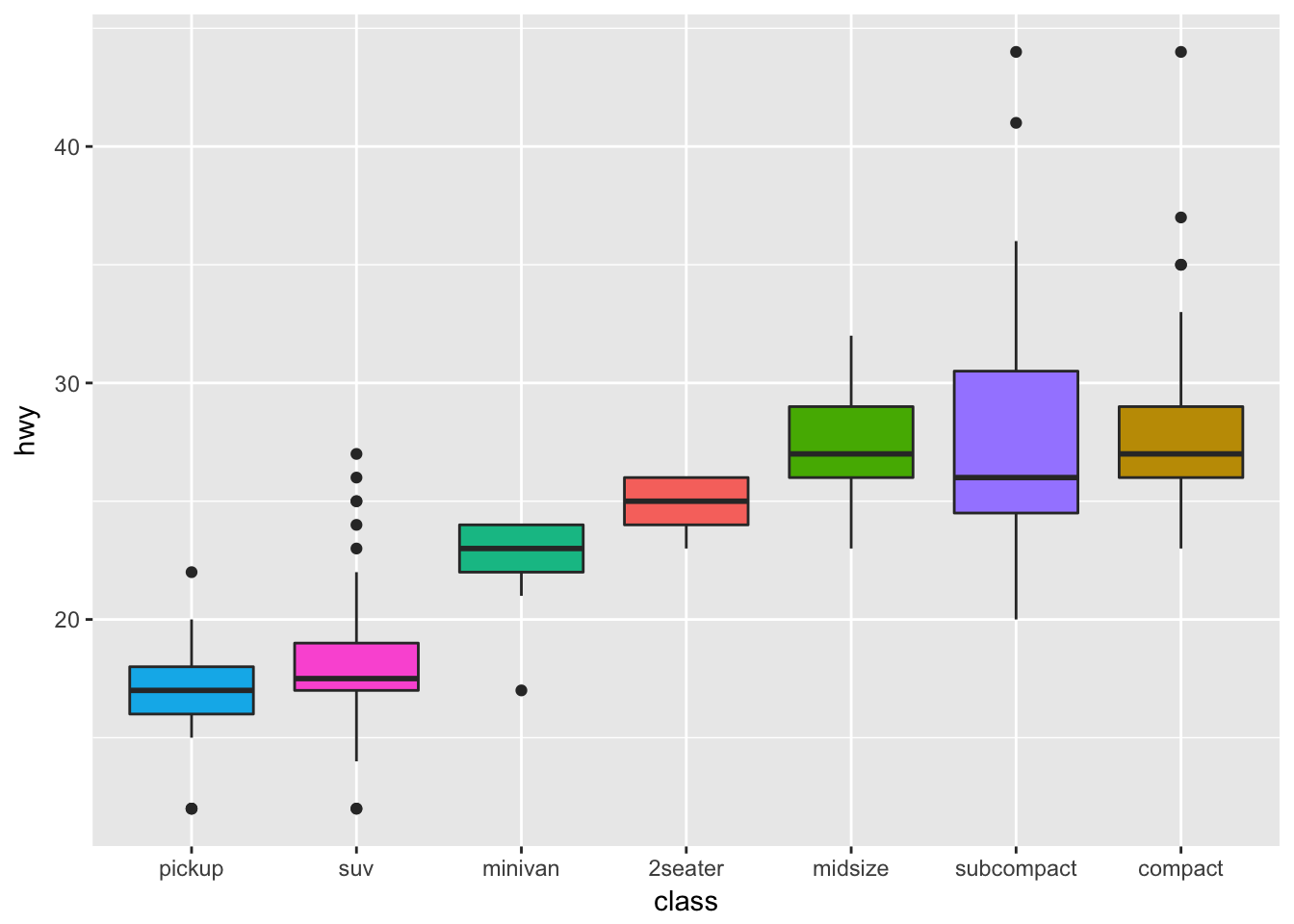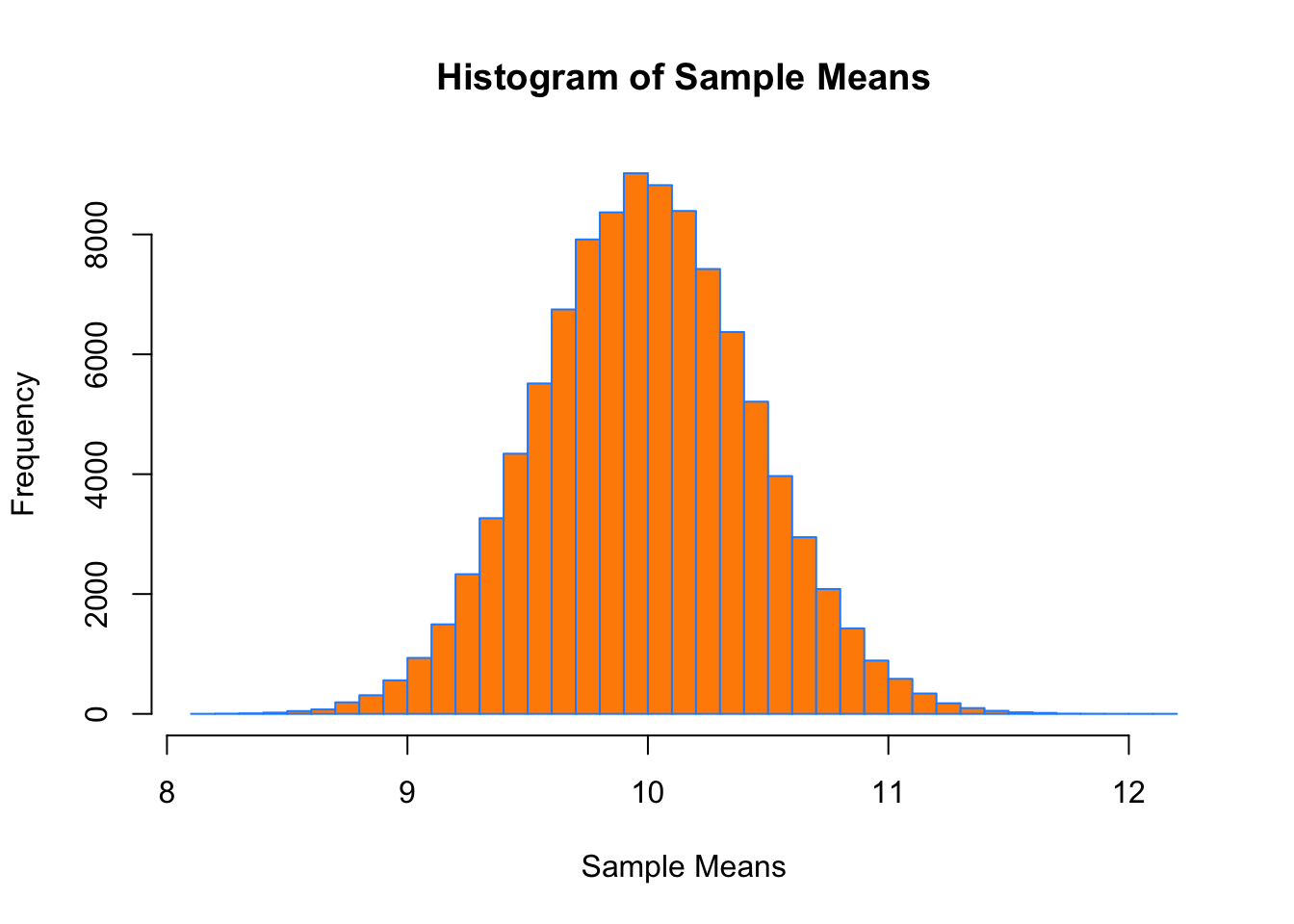# Basic Calculations

[Exercise] Calculate $$e^2$$.

exp(2)
##  7.389056

[Exercise] Calculate the natural log of 3.

log(3)
##  1.098612

# Getting Help

y = c(0, 2, NA, 3, 4, 1, 9, 0)

[Exercise] Calculate the mean of y with the missing values removed. Use only the mean() function.

• Hint 1: Check the documentation for mean().
• Hint 2: If you’re really stuck, there’s always this approach.
mean(y, na.rm = TRUE)
##  2.714286

# Packages

[Exercise] Run the following code:

ggplot(mpg, aes(x = reorder(class, hwy), y = hwy, fill = class)) +
geom_boxplot() +
xlab("class") +
theme(legend.position = "none")

To do so, you will need to make sure the ggplot2 package is installed, and loaded.

# install.packages("ggplot2")
library(ggplot2)
ggplot(mpg, aes(x = reorder(class, hwy), y = hwy, fill = class)) +
geom_boxplot() +
xlab("class") +
theme(legend.position = "none")[Exercise] Modify the following code to run, but without loading the entire MASS library. The lda() function is from the MASS package.

MASS::lda(Species ~ ., data = iris)\$means
##            Sepal.Length Sepal.Width Petal.Length Petal.Width
## setosa            5.006       3.428        1.462       0.246
## versicolor        5.936       2.770        4.260       1.326
## virginica         6.588       2.974        5.552       2.026

# Vectors and Lists

x = 1:100

[Exercise] Calculate

$\sum_{i = 1}^{n} \ln(x_i).$

That is, sum the natural log of each element of x.

sum(log(x))
##  363.7394

[Exercise] After running the following code, how many of the elements of some_vector are larger than 1? A good solution will use only one line of code.

set.seed(42)
some_vector = rnorm(100)
sum(some_vector > 1)
##  17

# Probability

[Exercise] Consider a random variable $$X$$ that has a normal distribution with a mean of 5 and a variance of 9. Calculate

$P[X > c],$

for $$c = 1, 2, 3, 4, 5, 6, 7, 8, 9, 10.$$

c = 1:10
pnorm(c, mean = 5, sd = 3, lower.tail = FALSE)
##   0.90878878 0.84134475 0.74750746 0.63055866 0.50000000 0.36944134
##   0.25249254 0.15865525 0.09121122 0.04779035

# Style

The following code is “correct” but terrible. Don’t write code like this!

set.seed(1337);mu=10;sample_size=50;samples=100000;
x_bars=rep(0, samples)
for(i in 1:samples)
{
x_bars[i]=mean(rpois(sample_size,lambda = mu))}
x_bar_hist=hist(x_bars,breaks=50,main="Histogram of Sample Means",xlab="Sample Means",col="darkorange",border = "dodgerblue")
mean(x_bars>mu-2*sqrt(mu)/sqrt(sample_size)&x_bars<mu+2*sqrt(mu)/sqrt(sample_size))

[Exercise] Fix this code! You don’t need to change how the code accomplishes the task, but you should update the style.

set.seed(1337)
mu = 10
sample_size = 50
samples = 100000
x_bars = rep(0, samples)

for (i in 1:samples) {
x_bars[i] = mean(rpois(sample_size, lambda = mu))
}

x_bar_hist = hist(x_bars,
breaks = 50,
main = "Histogram of Sample Means",
xlab = "Sample Means",
col = "darkorange",
border = "dodgerblue")mean(x_bars > mu - 2 * sqrt(mu) / sqrt(sample_size) &
x_bars < mu + 2 * sqrt(mu) / sqrt(sample_size))
##  0.95429

Some things that were “wrong” with that code:

• Line length too long.
• Use of ; is discouraged.
• Opening { should be on same lines as for()
• Lack of indentation.
• No spaces after each ,
• No spaces around binary operators.